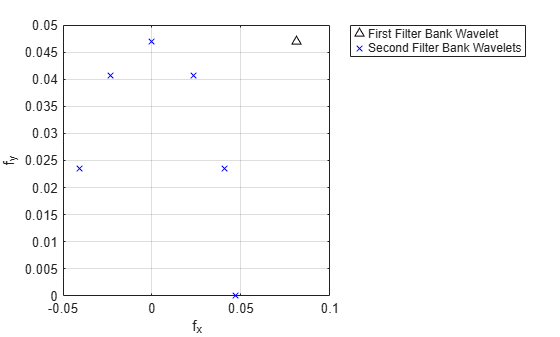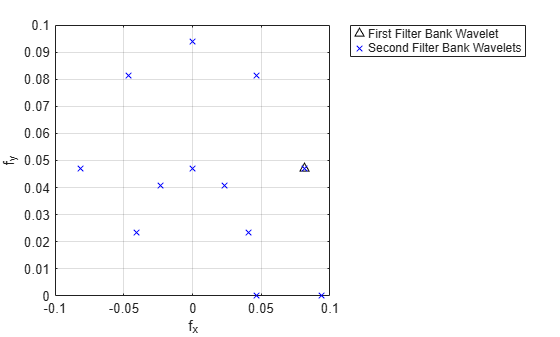# paths

Scattering paths

## Syntax

``spaths = paths(sf)``
``[spaths,npaths] = paths(sf)``

## Description

````spaths = paths(sf)` returns the scattering paths for all orders of the scattering network, `sf`. `spaths` is a cell array of MATLAB® tables with n elements, where n is the number of orders in the scattering network.```

example

````[spaths,npaths] = paths(sf)` returns the number of paths in each order as n-by-1 column vector, where n is the number of orders in the scattering network. The sum of the elements of `npaths` is the total number of scattering paths.```

## Examples

collapse all

Create an image scattering network with an image size of 256-by-256 and invariance scale equal to the minimum of the image size. The default `OptimizePath` value is 1 (`true`).

`sf = waveletScattering2('ImageSize',[256 256],'InvarianceScale',128)`
```sf = waveletScattering2 with properties: ImageSize: [256 256] InvarianceScale: 128 NumRotations: [6 6] QualityFactors: [1 1] Precision: "single" OversamplingFactor: 0 OptimizePath: 1 ```

Obtain the number of scattering paths in each order. Display the total number of scattering paths.

```[spaths,npaths] = paths(sf); sum(npaths)```
```ans = 391 ```

Set the `OptimizePath` value of the network to `false`. Display the total number of scattering paths. For the modified network, the scattering transform does not reduce the number of paths to compute based on a bandwidth consideration.

```sf.OptimizePath = false; [spaths,npaths] = paths(sf); sum(npaths)```
```ans = 571 ```

This example shows how the `OptimizePath` property can affect the scattering paths that include a specific wavelet.

Create the default wavelet image scattering network. Obtain all the wavelet filters and center spatial frequencies for the network. Obtain all the scattering paths. Display the total number of paths.

```sf = waveletScattering2 [~,psifilters,f] = filterbank(sf); [spaths,npaths] = paths(sf); disp(['Total Number of Paths: ',num2str(sum(npaths))]) ```
```sf = waveletScattering2 with properties: ImageSize: [128 128] InvarianceScale: 64 NumRotations: [6 6] QualityFactors: [1 1] Precision: 'single' OversamplingFactor: 0 OptimizePath: 1 Total Number of Paths: 241 ```

Display the number of wavelet filters in each filter bank.

```disp(['Filter Bank 1: ',num2str(size(psifilters{1},3))]); disp(['Filter Bank 2: ',num2str(size(psifilters{2},3))]); ```
```Filter Bank 1: 24 Filter Bank 2: 24 ```

Choose a wavelet from the first filter bank and display its spatial center frequency. Use `spaths` to find all the three-element paths that include the chosen wavelet. Display the paths.

```waveletA = 14; disp(['Center Frequency: ',num2str(f{1}(waveletA,:))]); ind = find(spaths{3}.path(:,2)==waveletA); spaths{3}(ind,:) ```
```Center Frequency: 0.08119 0.046875 ans = 6x1 table path _____________ 0 14 19 0 14 20 0 14 21 0 14 22 0 14 23 0 14 24 ```

Plot the center frequencies of the wavelet filters on the paths.

```plot(f{1}(waveletA,1),f{1}(waveletA,2),'k^'); xlabel('f_x') ylabel('f_y') hold on waveletBs = spaths{3}.path(ind,3); plot(f{2}(waveletBs,1),f{2}(waveletBs,2),'bx'); grid on legend('First Filter Bank Wavelet','Second Filter Bank Wavelets',... 'Location','northeastoutside') ```Now set the `OptimizePath` property of the scattering network `sf` to `false`. Obtain the wavelet filters, center spatial frequencies, and scattering paths of the network.

```sf.OptimizePath = false [~,psifilters2,f2] = filterbank(sf); [spaths2,npaths2] = paths(sf); disp(['Total Number of Paths: ',num2str(sum(npaths2))]) ```
```sf = waveletScattering2 with properties: ImageSize: [128 128] InvarianceScale: 64 NumRotations: [6 6] QualityFactors: [1 1] Precision: 'single' OversamplingFactor: 0 OptimizePath: 0 Total Number of Paths: 385 ```

Choose the same wavelet as above. To confirm it is the same wavelet, display its spatial center frequency. Use `spaths` to find all the three-element paths that include the wavelet. Because `OptimizePath` is set to `false`, the wavelet filter has more children.

```waveletA = 14; disp(['Center Frequency: ',num2str(f2{1}(waveletA,:))]); ind = find(spaths2{3}.path(:,2)==waveletA); spaths2{3}(ind,:) ```
```Center Frequency: 0.08119 0.046875 ans = 12x1 table path _____________ 0 14 13 0 14 14 0 14 15 0 14 16 0 14 17 0 14 18 0 14 19 0 14 20 0 14 21 0 14 22 0 14 23 0 14 24 ```

Plot the center frequencies of the wavelet filters on the paths. Some of child filters have center frequencies higher than the chosen wavelet.

```figure plot(f2{1}(waveletA,1),f2{1}(waveletA,2),'k^'); xlabel('f_x') ylabel('f_y') hold on waveletBs = spaths2{3}.path(ind,3); plot(f2{2}(waveletBs,1),f2{2}(waveletBs,2),'bx'); grid on legend('First Filter Bank Wavelet','Second Filter Bank Wavelets',... 'Location','northeastoutside') ```## Input Arguments

collapse all

Wavelet image scattering network, specified as a `waveletScattering2` object.

## Output Arguments

collapse all

Scattering paths of all orders of the scattering network, returned as a cell array of MATLAB tables. `spaths` has n elements, where n is the number of orders in the scattering network.

Each MATLAB table in `spaths` contains a single variable, `path`. The variable `path` is a row vector with one column for each element of the path. The scalar 0 denotes the original image. Positive integers in the Lth column denote the corresponding wavelet filter in the (L−1)th filter bank. Wavelet bandpass filters are ordered by decreasing center frequency. There are `NumRotations` wavelets per center frequency pair.

Number of scattering paths in each order of the scattering network. `npaths` is a no-by-1 column vector where no is the number of orders in the scattering network. The sum of the elements of `npaths` is the total number of scattering paths.

## Version History

Introduced in R2019a